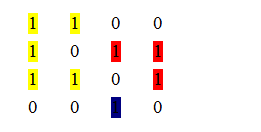Q:

# Find the number of islands

A group of connected 1's forms an island. There is a matrix of N×M. You have to find out the numbers of item.

Example:

If there is a matrix:Here three groups ones are possible. One is red one is yellow and one is blue.
Output: 3

Algorithm:

To solve this problem we follow this approach:

1. We take a stack and whenever we find the first 1 we push the position into the stack and make that position visited.
2. Take the first element from the stack and pop from the stack.
3. Check it's four neighbors. If out of them if anyone is 1 then we also push the position into the stack.
4. Repeat the process until the queue is empty.
5. Count the numbers when the queue is empty and its give the island count.

## C++ Implementation to find the number of islands

``````#include <bits/stdc++.h>
using namespace std;

int x[] = { -1, 1, 0, 0 };
int y[] = { 0, 0, -1, 1 };
//check the validity of the position
//with respect to the matrix.
bool isvalid(int x_axis, int y_axis, int n)
{
return (x_axis >= 0 && x_axis < n && y_axis >= 0 && y_axis < n);
}
//function to count the no. of Island
int Island_count(int* arr, int n)
{
int count = 0;
stack<pair<int, int> > s;
set<pair<int, int> > st;
for (int i = 0; i < n; i++) {
for (int j = 0; j < n; j++) {
if (*(arr + i * n + j) == 1 && st.find(make_pair(i, j)) == st.end()) {
//push the position with value 1 into the stack
s.push(make_pair(i, j));
//make the position visited
st.insert(make_pair(i, j));
while (!s.empty()) {
//take the top element of the stack
pair<int, int> p = s.top();
s.pop();
//check it's neighbours
for (int i = 0; i < 4; i++) {
int x_axis = p.first + x[i];
int y_axis = p.second + y[i];
//if the position is valid and it is not visited
//then push that position into stack
if (isvalid(x_axis, y_axis, n) && st.find(make_pair(x_axis, y_axis)) == st.end() && *(arr + x_axis * n + y_axis) == 1) {
s.push(make_pair(x_axis, y_axis));
st.insert(make_pair(x_axis, y_axis));
}
}
}
//every time when stack is empty means
//we visited all the group of 1's
count++;
}
}
}
return count;
}

int main()
{
int size = 4;
int arr[size][size] = { { 1, 1, 0, 0 }, { 1, 0, 1, 1 }, { 1, 1, 0, 1 }, { 0, 0, 1, 0 } };
cout << "No. Of Island is : ";
cout << Island_count(&arr, size) << endl;
return 0;
}
``````

Output

`The No. of Island is : 3`